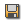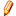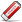Understanding Cavalieri's Principle (T)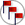[no district selected]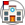[no school selected]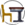[no class selected]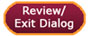Dialog Completion1 of 18
Purpose of this Instructional Dialog
 Mathematical understanding and procedural skill are equally important, and both are assessable using mathematical tasks of sufficient richness. One hallmark of mathematical understanding is the ability to justify, in a way appropriate to the student’s mathematical maturity, why a particular mathematical statement is true or where a mathematical rule comes from. (from the introduction to the Common Core State Standards for Mathematics) Phrases such as "Know formulas for...," "Find the volume...," and "Apply the concept of..." are easy to find in most mathematics frameworks, while words such as "derive," "explain," "illustrate," and "verify" in reference to geometric formulas are rare. Common Core addresses this disparity by including standards that are entirely devoted to the understanding of such formulas. Two of these are standards G-GMD.1 and G-GMD.2, where students are required to explain the volume formulas that they have learned to recite, write, and apply. Mastery of these standards requires students to be familiar with Cavalieri's principle, which is the focus here. The goal is to guide students toward an understanding of Cavalieri's principle, using what they intuitively understand about right prisms as a starting point. To accomplish this, students and teachers are led through a series of "concept-building questions." Some of these questions are designed to elicit simple responses; however, most require students to formulate explanations based on what they know and understand—a skill common in Depth of Knowledge levels 2-4.